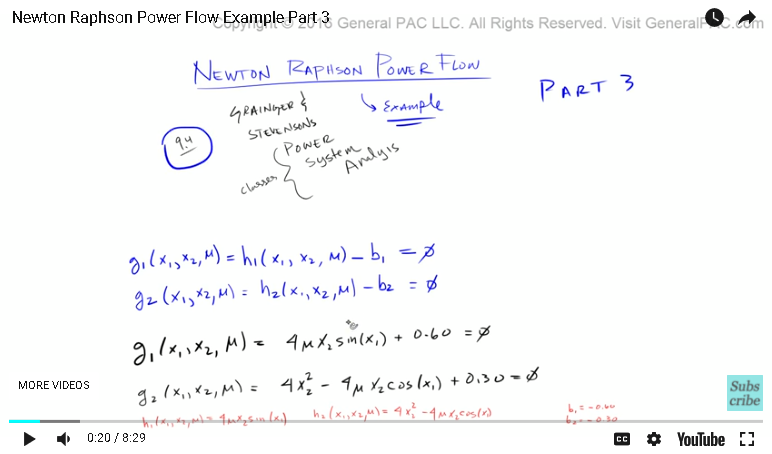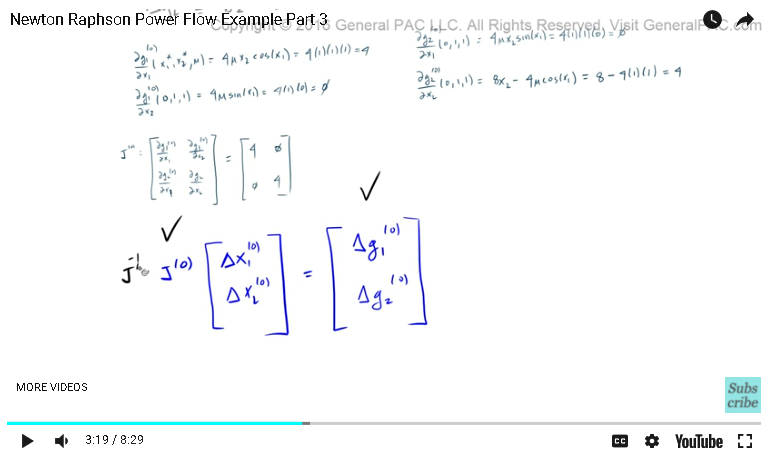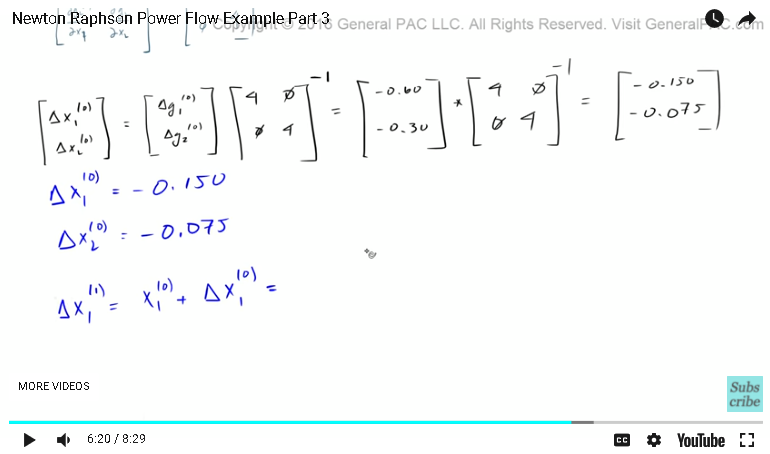This video was brought to you by GeneralPAC.com, making power systems Intuitive, Open and Free for Everyone, Everywhere. Consider subscribing and supporting through patreon.com/GeneralPAC. This is a mechanism for you to support us financially so we can continue making high quality power system video tutorials. Our corporate sponsor for this topic is AllumiaX.com from Seattle, Washington. Contact them for industrial and commercial power system studies.

Okay. So this part three of the tutorial where we cover an example of the Newton Raphson Power Flow Method. Okay? And this example comes from the Grainger and Stevenson's Power System Analysis book. This is example 9.4 in section 9.3Okay. So we kind of began this series by introducing the equations that were presented in this book. And we said, "Well, don't worry about these equations yet. Just follow along and look at how this method is done overall."

We took the partial derivative of these equations, with respect to x1 and x2. We took the ... we determined the Jacobian matrix and we said, "Well, the Jacobian matrix is simply a matrix of the partial derivatives."

We had some initial values. And these initial values were presented in the book. And we used these initial values to determine our first initial mismatch. And we also used the initial values to determine our zero-ith iteration for the Jacobian matrix, which is basically plugging in the initial values into the partial derivatives and determining the results.

Okay. Now, let's get into the exciting part. Now, the exciting part is that we already have this Jacobian matrix, right? So if we take this Jacobian matrix, right, for the zero-ith iteration and we multiply this Jacobian matrix by our initial values of Dx1 and Dx2, of the zero-ith iteration, we will get this right here, or a mismatch.

So Dg1 of the zero-ith value and Dg2 of the zero-ith value. Okay? So this is essentially the meat of the Newton Raphson Method. Now, what we have is this, right? We have this term there. We have the Jacobian matrix and we have the Jacobian matrix for the zero-ith iteration, which is just plugging in our initial values. Right?

And we also have these terms right here. We have Delta g1 and Delta g2, or our mismatch, for the initial values. But what we don't have is the change of x1 for the zero-ith iteration and the change of x2 for the zero-ith iteration. So we have this guy, we have this guy. We have to find what this ... essentially, we have to figure out what Delta x1 is and Delta x2 is.

Well, if we solve this like a basic linear algebra problem, how would we do it? Well, it's pretty straightforward, right? What if we just multiply both side of these equations by the inverse of the Jacobian matrix? So what if we take the Jacobian matrix, find the inverse of the Jacobian matrix, and we multiply that to both sides of the equation. Right?And if we had done that, we know by linear algebra that A times the inverse, well, that's just going to equal 1 or the unity matrix. That's going to simply equal 1. And the only thing that's going to the left on the left-hand side of the equations are these guys there. And the right side of the equation, well, we already knew what this value is. And we could determine the inverse of the Jacobian matrix here.

So that brings us to the next set of equations, which is Delta x1 ... I mean, Delta x2 of the zero-ith iteration. Those guys are equal to Delta g1 of the zero-ith and Delta g2 of the zero-ith. Multiply that by ... remember the Jacobian matrix, we said was 4, 0, 0, and 4. But now we have to take the inverse of this Jacobian matrix.

So now, let's put these values in, right? So Delta g1, Delta g1, I put a zero-ith iteration is equal to -0.60. Delta g2 for the zero-ith iteration is equal to -.130. -0.60, -0.30, multiply that. Okay. Inverse.

Now according to the book, if we take this matrix here and we multiply by the inverse of this matrix there, then we should get a value of -0.150 and -0.075. Okay. Let's look at what we actually solved for.

So we said that the change of x1 for the zero-ith iteration is equal to -0.150. And the change of x2 for the zero-ith iteration is equal to -.075. Okay? Which, also, by the way, matches the book, because that's where I get these answers from. Now, that's not your answer. That's just a part of the answer, is that we have the basic building blocks for the next set of iterations.

So the next iteration, we have to do the same exact process all over again, is ... is this. We have Delta x1, but now we have the next set of iteration. That's why we advance the superscript to 1. That is equal to x1 of the zero-ith plus Delta x1 of the zero-ith. We know what x1 of the zero-ith was. Well, that was just simply our initial condition. Right? And which our initial condition, if you all remember, was 0.So our initial condition of x1 was just equal to 0. So that is gonna equal 0 plus Delta x1, which we calculated as -0.150, which means the next set of iteration is -.150. Okay? And same thing with x2 for the next iteration, is equal to x1 for the next ... the zero-ith plus Delta x2 to the zero-ith. And that is equal to ... well, x1 ... sorry. This is x2. So x2 for the zero-ith, we said that that was equal to 1, if you remember correctly. On x1, right here. X2 is equal to 1, right?

So go back over here. We know that x2 was equal to 1, plus now the change of x2, which we said is this guy right here, is equal to -0.075. And that is gonna equal 0.925. All right.

So now, we have the building blocks for the second iteration. So I'm gonna highlight that here. Now we have the building blocks for the second.

And this will conclude part three. Now, if you haven't already, please go ahead and subscribe by clicking on the bottom right corner of the screen. And if you have questions, there's a link at the bottom of this page for a Q&A forum. And in this forum, you can go and ask as many questions as you like. And hopefully, the community will respond to your questions so that we all can learn and understand this stuff more intuitively.

This video was brought to you by GeneralPAC.com, making power systems intuitive.

#### Greetings from the GeneralPAC Team!

We make high-quality Power Systems Video Tutorials on complex topics that are free and open to everyone!  Thank you so much for supporting us through Patreon so we can continue doing good and valuable work.

What is Patreon and why do we use it?

Patreon is a fantastic portal that allows our fans and community to make monthly contribution (like Netflix subscription) so we can continue creating high-quality power systems video tutorials. In return, you get access to incredible perks like voting on future topics, getting your questions answered, access to VIP Q/A webinars with the creators of GeneralPAC, and much more! We THANK YOU for supporting us

Why do we need your support?

An incredible amount of time and effort is needed to develop high-quality video tutorials. Each video (Part 1 for example) takes approximately 10 hours to complete which includes learning the concept ourselves, brainstorming creative ways to teach and explain the concepts, writing the script, audio recording, video recording, and editing. It's no wonder why Hundreds-of-Thousands of people have watched, liked, subscribed, and left positive comments on Youtube channel. Your support truly makes all the difference.